# Parabola focus and directrix

By Martin McBride, 2023-01-29
Tags: parabola locus focus directrix
Categories: coordinate systems pure mathematicsWe have previously seen how a parabola is defined in terms of parametric equations or alternatively in Cartesian form.

An alternative way to define a parabola is as a locus of points.

Here is a video:

## Focus and directrix

The locus defining a parabola depends on a focus and a directrix.

The focus is a point. For a standard parabola, the focus is located on the x-axis at a distance a from the origin, that is at the point (a, 0). a is the constant in the parabola equation: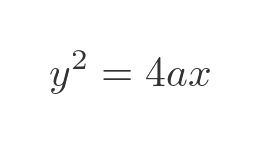The directrix is a line. For a standard parabola, it is a line perpendicular to the x-axis passing through (-a, 0), that is the line x = -a.

The vertex of the parabola is its turning point. If we draw a perpendicular line between the focus and directrix, the vertex is the midpoint of that line. This is always at the origin for a standard parabola.

This diagram shows the focus, directrix and vertex: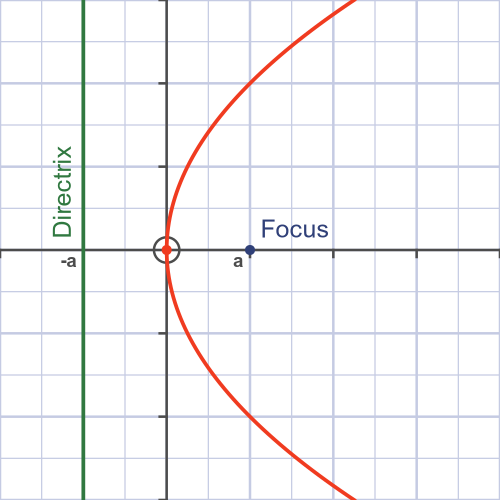## The locus

A parabola is the locus of all points P where the perpendicular distance from the directrix is equal to the distance from the focus.:This is illustrated here:The animation below also illustrates this: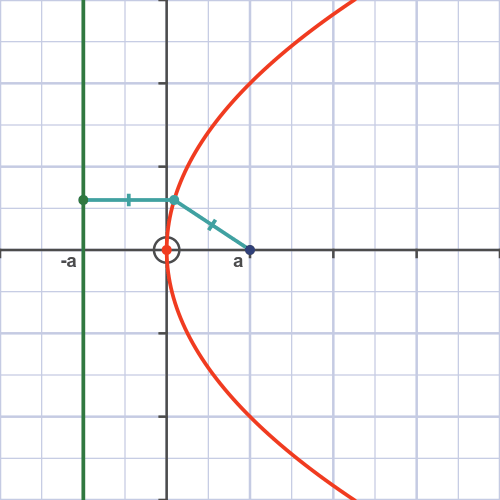## Proof that locus is a parabola

We can prove the locus above creates a parabola.

The three points of interest are:

• F, the focus, which is at (a, 0).
• D, any point on the directrix, which will have coordinates (-a, y).
• P, the point (x, y) where FP equals PD.

First, we can find the length FP. We use that fact that the distance l between two points (x0, y0) and (x1, y1) can be found from Pythagoras' theorem:Substituting the coordinates of F and P gives: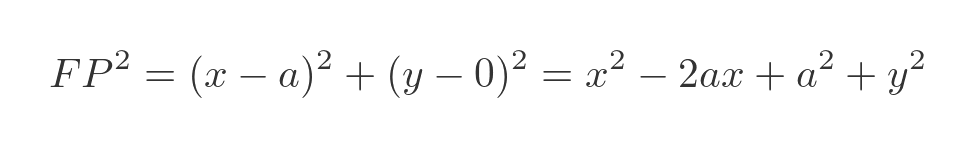Next, we will find PD. The directrix is vertical, so PD must be horizontal since it is the perpendicular distance. So the distance between P and D is simply the horizontal distance:It will be useful to find the square of PD:The locus is all points where FP equals PD. We can find this by equating the squares of FP and PD, which we have just calculated. This gives us an equation that relates x and y for every point on the curve: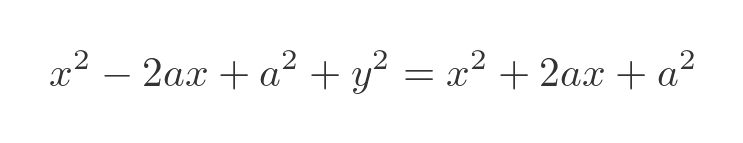Cancelling the terms in x squared and a squared, and simplifying, gives:This gives a formula for x as a function of y: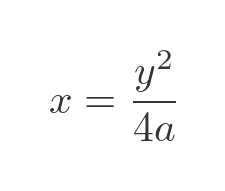This is the Cartesian equation for a parabola we saw in the main parabolas article.

## Directrix parallel to the x-axis

We can use a similar locus to construct a parabola with the directrix parallel to the x-axis. To do this we must also move the focus to point (0, a). Here is the new locus: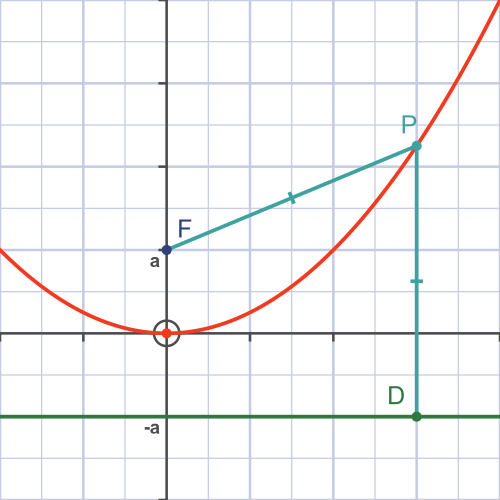We can find the Cartesian equation of this curve by swapping x and y in the previous equation: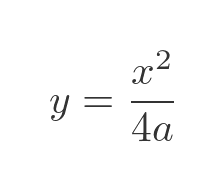## Locus of a general quadratic equation

A parabola, of course, is just a quadratic curve. But so far we have only drawn curves where the vertex is at the origin. In this section we will see how to find the locus of any quadratic function.

We can translate any curve y = f(x) by substituting: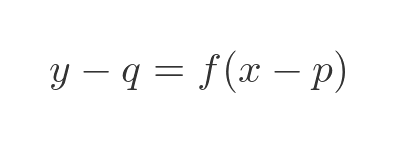This will shift the curve by p in the x direction and by q in the y direction.

Applying this to the parabola formula: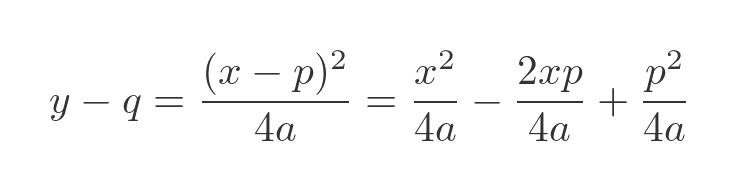Which gives us the quadratic equation: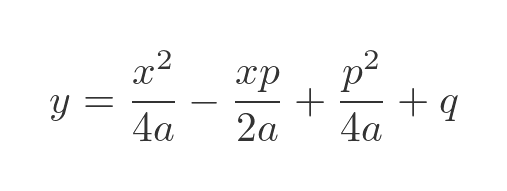Here is a graph for a = 1, p = 1 and q = 2:With suitable values for a, p and q, this can be used to create any quadratic graph.